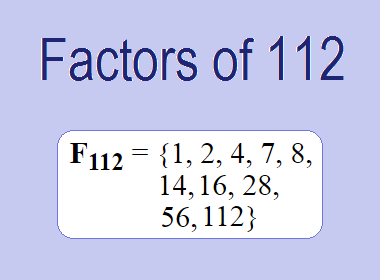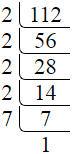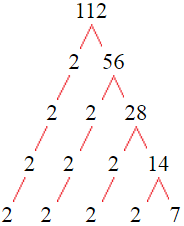# Factors of 112The factors of 112 are 1, 2, 4, 7, 8, 14, 16, 28, 56, and 112 i.e. F112 = {1, 2, 4, 7, 8, 14, 16, 28, 56, 112}. The factors of 112 are all the numbers that can divide 112 without leaving a remainder.

We can check if these numbers are factors of 112 by dividing 112 by each of them. If the result is a whole number, then the number is a factor of 112. Let's do this for each of the numbers listed above:

·        1 is a factor of 112 because 112 divided by 1 is 112.

·        2 is a factor of 112 because 112 divided by 2 is 56.

·        4 is a factor of 112 because 112 divided by 4 is 28.

·        7 is a factor of 112 because 112 divided by 7 is 16.

·        8 is a factor of 112 because 112 divided by 8 is 14.

·        14 is a factor of 112 because 112 divided by 14 is 8.

·        16 is a factor of 112 because 112 divided by 16 is 7.

·        28 is a factor of 112 because 112 divided by 28 is 4.

·        56 is a factor of 112 because 112 divided by 56 is 2.

·        112 is a factor of 112 because 112 divided by 112 is 1.

## How to Find Factors of 112?

1 and the number itself are the factors of every number. So, 1 and 112 are two factors of 112. To find the other factors of 112, we can start by dividing 112 by the numbers between 1 and 112. If we divide 112 by 2, we get a remainder of 0. Therefore, 2 is a factor of 112. If we divide 112 by 3, we get a remainder of 1. Therefore, 3 is not a factor of 112.

Next, we can check if 4 is a factor of 112. If we divide 112 by 4, we get a remainder of 0. Therefore, 4 is also a factor of 112. We can continue this process for all the possible factors of 112.

Through this process, we can find that the factors of 112 are 1, 2, 4, 7, 8, 14, 16, 28, 56, and 112. These are the only numbers that can divide 112 without leaving a remainder.

********************

********************

## Properties of the Factors of 112

The factors of 112 have some interesting properties. One of the properties is that the sum of the factors of 112 is equal to 248. We can see this by adding all the factors of 112 together:

1 + 2 + 4 + 7 + 8 + 14 + 16 + 28 + 56 + 112 = 248

Another property of the factors of 112 is that the prime factors of 112 are 2, and 7 only.

## Applications of the Factors of 112

The factors of 112 have several applications in mathematics. One of the applications is in finding the highest common factor (HCF) of two or more numbers. The HCF is the largest factor that two or more numbers have in common. For example, to find the HCF of 112 and 120, we need to find the factors of both numbers and identify the largest factor they have in common. The factors of 112 are 1, 2, 4, 7, 8, 14, 16, 28, 56, and 112. The factors of 120 are 1, 2, 3, 5, 6, 8, 10, 12, 15, 20, 24, 30, 40, 60, and 120. The largest factor that they have in common is 8. Therefore, the HCF of 112 and 120 is 8.

Another application of the factors of 112 is in prime factorization. Prime factorization is the process of expressing a number as the product of its prime factors. The prime factors of 112 are 2, and 7, since these are the only prime numbers that can divide 112 without leaving a remainder. Therefore, we can express 112 as:

112 = 2 × 2 × 2 × 2 × 7

We can do prime factorization by division and factor tree method also. Here is the prime factorization of 112 by division method,112 = 2 × 2 × 2 × 2 × 7

Here is the prime factorization of 112 by the factor tree method,112 = 2 × 2 × 2 × 2 × 7

## Conclusion

The factors of 112 are the numbers that can divide 112 without leaving a remainder. The factors of 112 are 1, 2, 4, 7, 8, 14, 16, 28, 56, and 112. The factors of 112 have some interesting properties, such as having a sum of 248. The factors of 112 have several applications in mathematics, such as finding the highest common factor and prime factorization.